Modified Euler's method for ordinary difference equation using Mathematica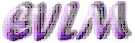Anotation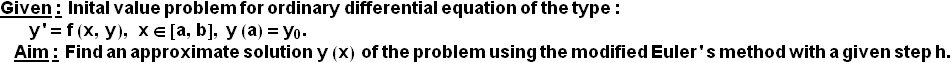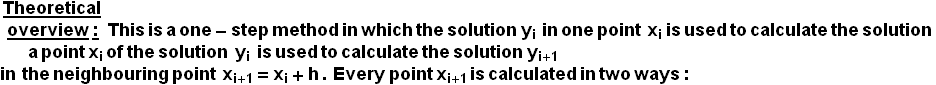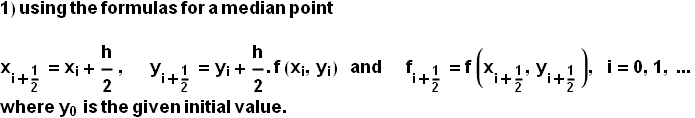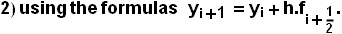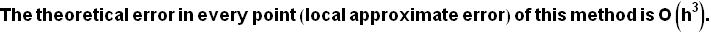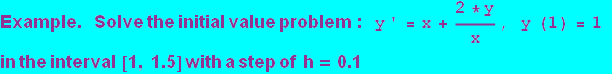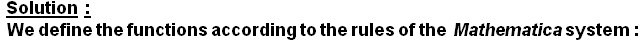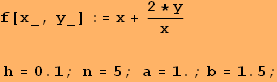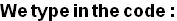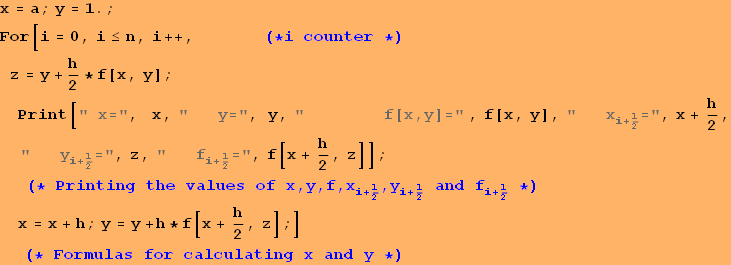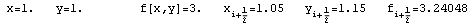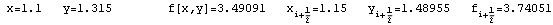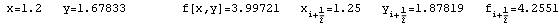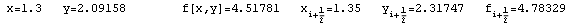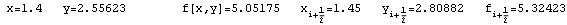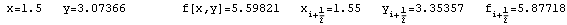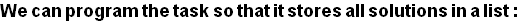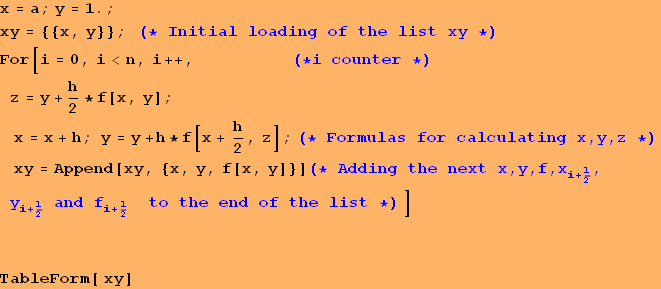1 1 1.1 1.315 3.49091 1.2 1.67833 3.99721 1.3 2.09158 4.51781 1.4 2.55623 5.05175 1.5 3.07366 5.59821

Conclusion:  The solution derived using the modified Euler's method is in the form of a table of the variable function y(x), as shown in the second column of the table above. Since the global apriori error of the method is О(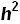) and here h=0.1, then these solutions must be rounded to only two signs after the decimal point.

Graphic of the solution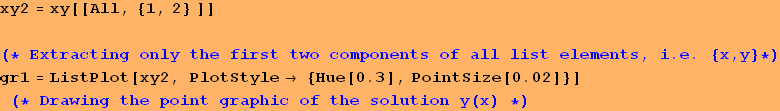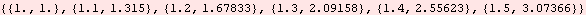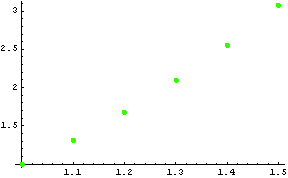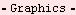Created by Wolfram Mathematica 6.0  (22 September 2008)